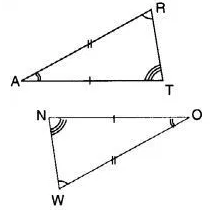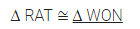# In the figure, the two triangles are congruent. The corresponding parts are marked. We can write ∆ RAT =?

In the figure, the two triangles are congruent. The corresponding parts are marked. We can write ∆ RAT = ?